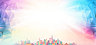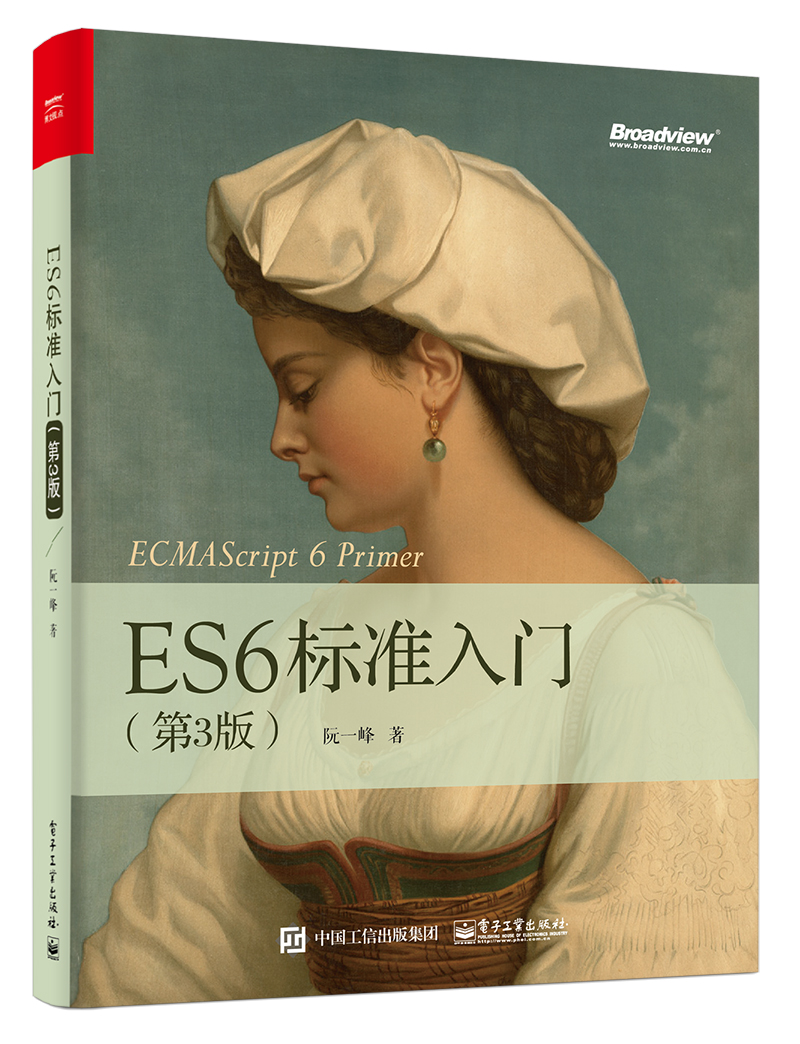# ECMAScript6编码风格

• 资源类别： JavaScript
• 如遇问题：请底部留言反馈或联系客服## 块级作用域

（1）let 取代 var

ES6提出了两个新的声明变量的命令：`let``const`。其中，`let`完全可以取代`var`，因为两者语义相同，而且`let`没有副作用。

``````'use strict';

if (true) {
let x = 'hello';
}

for (let i = 0; i < 10; i++) {
console.log(i);
}``````

`var`命令存在变量提升效用，`let`命令没有这个问题。

``````'use strict';

if(true) {
console.log(x); // ReferenceError
let x = 'hello';
}``````

（2）全局常量和线程安全

`let``const`之间，建议优先使用`const`，尤其是在全局环境，不应该设置变量，只应设置常量。

`const`优于`let`有几个原因。一个是`const`可以提醒阅读程序的人，这个变量不应该改变；另一个是`const`比较符合函数式编程思想，运算不改变值，只是新建值，而且这样也有利于将来的分布式运算；最后一个原因是 JavaScript 编译器会对`const`进行优化，所以多使用`const`，有利于提供程序的运行效率，也就是说`let``const`的本质区别，其实是编译器内部的处理不同。

``````// bad
var a = 1, b = 2, c = 3;

// good
const a = 1;
const b = 2;
const c = 3;

// best
const [a, b, c] = [1, 2, 3];``````

`const`声明常量还有两个好处，一是阅读代码的人立刻会意识到不应该修改这个值，二是防止了无意间修改变量值所导致的错误。

## 字符串

``````// bad
const a = "foobar";
const b = 'foo' + a + 'bar';

// acceptable
const c = foobar;

// good
const a = 'foobar';
const b = foo\${a}bar;
const c = 'foobar';``````

## 解构赋值

``````const arr = [1, 2, 3, 4];

const first = arr;
const second = arr;

// good
const [first, second] = arr;``````

``````// bad
function getFullName(user) {
const firstName = user.firstName;
const lastName = user.lastName;
}

// good
function getFullName(obj) {
const { firstName, lastName } = obj;
}

// best
function getFullName({ firstName, lastName }) {
}``````

``````// bad
function processInput(input) {
return [left, right, top, bottom];
}

// good
function processInput(input) {
return { left, right, top, bottom };
}

const { left, right } = processInput(input);``````

## 对象

``````// bad
const a = { k1: v1, k2: v2, };
const b = {
k1: v1,
k2: v2
};

// good
const a = { k1: v1, k2: v2 };
const b = {
k1: v1,
k2: v2,
};``````

``````// bad
const a = {};
a.x = 3;

// if reshape unavoidable
const a = {};
Object.assign(a, { x: 3 });

// good
const a = { x: null };
a.x = 3;``````

``````// bad
const obj = {
id: 5,
name: 'San Francisco',
};
obj[getKey('enabled')] = true;

// good
const obj = {
id: 5,
name: 'San Francisco',
[getKey('enabled')]: true,
};``````

``````var ref = 'some value';

const atom = {
ref: ref,

value: 1,

return atom.value + value;
},
};

// good
const atom = {
ref,

value: 1,

return atom.value + value;
},
};``````

## 数组

``````// bad
const len = items.length;
const itemsCopy = [];
let i;

for (i = 0; i < len; i++) {
itemsCopy[i] = items[i];
}

// good
const itemsCopy = [...items];``````

``````const foo = document.querySelectorAll('.foo');
const nodes = Array.from(foo);``````

## 函数

``````(() => {
console.log('Welcome to the Internet.');
})();``````

``````// bad
[1, 2, 3].map(function (x) {
return x * x;
});

// good
[1, 2, 3].map((x) => {
return x * x;
});

// best
[1, 2, 3].map(x => x * x);``````

``````// bad
const self = this;
const boundMethod = function(...params) {
return method.apply(self, params);
}

// acceptable
const boundMethod = method.bind(this);

// best
const boundMethod = (...params) => method.apply(this, params);``````

``````// bad
function divide(a, b, option = false ) {
}

// good
function divide(a, b, { option = false } = {}) {
}``````

``````// bad
function concatenateAll() {
const args = Array.prototype.slice.call(arguments);
return args.join('');
}

// good
function concatenateAll(...args) {
return args.join('');
}``````

``````// bad
function handleThings(opts) {
opts = opts || {};
}

// good
function handleThings(opts = {}) {
// ...
}``````

## Map结构

``````let map = new Map(arr);

for (let key of map.keys()) {
console.log(key);
}

for (let value of map.values()) {
console.log(value);
}

for (let item of map.entries()) {
console.log(item, item);
}``````

## Class

``````// bad
function Queue(contents = []) {
this._queue = [...contents];
}
Queue.prototype.pop = function() {
const value = this._queue;
this._queue.splice(0, 1);
return value;
}

// good
class Queue {
constructor(contents = []) {
this._queue = [...contents];
}
pop() {
const value = this._queue;
this._queue.splice(0, 1);
return value;
}
}``````

``````// bad
const inherits = require('inherits');
function PeekableQueue(contents) {
Queue.apply(this, contents);
}
inherits(PeekableQueue, Queue);
PeekableQueue.prototype.peek = function() {
return this._queue;
}

// good
class PeekableQueue extends Queue {
peek() {
return this._queue;
}
}``````

## 模块

``````// bad
const moduleA = require('moduleA');
const func1 = moduleA.func1;
const func2 = moduleA.func2;

// good
import { func1, func2 } from 'moduleA';``````

``````// commonJS的写法
var React = require('react');

render() {
return <nav />;
}
});

// ES6的写法
import React from 'react';

render() {
return <nav />;
}
});

``````// bad
import * as myObject './importModule';

// good
import myObject from './importModule';``````

``````function makeStyleGuide() {
}

export default makeStyleGuide;``````

``````const StyleGuide = {
es6: {
}
};

export default StyleGuide;``````

## ESLint的使用

ESLint是一个语法规则和代码风格的检查工具，可以用来保证写出语法正确、风格统一的代码。

``\$ npm i -g eslint``

``\$ npm i -g eslint-config-airbnb``

``````{
"extends": "eslint-config-airbnb"
}``````

`index.js`文件的代码如下。

``````var unusued = 'I have no purpose!';

function greet() {
var message = 'Hello, World!';
}

greet();``````

``````\$ eslint index.js
index.js
1:5  error  unusued is defined but never used                 no-unused-vars
4:5  error  Expected indentation of 2 characters but found 4  indent
5:5  error  Expected indentation of 2 characters but found 4  indent

✖ 3 problems (3 errors, 0 warnings)``````

## 超级好用的开发工具，程序员实用工具整理

2018-7-29 9:17:57

## 在vue项目中：统一封装 Axios 接口与异常处理

2018-8-9 11:01:56

0 条回复A文章作者M管理员
暂无讨论，说说你的看法吧